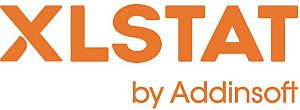# XLSTAT - Discretization of Quantitative Variables

## What is discretization?

Discretizing a numerical variable means transforming it into an ordinal variable.
This process is used in marketing where it is often referred to as segmentation.

## XLSTAT discretization tool

XLSTAT makes available several discretization methods that can be or not automatic.

• Constant range: Choose this method to create classes that have the same range. Then enter the value of the range. You can optionally specify the "minimum" that corresponds to the lower bound of the first interval.
• Intervals: Use this method to create a given number of intervals with the same range.
• Equal frequencies: Choose this method so that all the classes contain as much as possible the same number of observations.
• Automatic (Fisher): Use this method to create the classes using the Fisher’s algorithm.
• Automatic (k-means): Choose this method to create classes (or intervals) using the k-means algorithm.
• Intervals (user defined): Choose this option to select a column containing in increasing order the lower bound of the first interval, and the upper bound of all the intervals.
• 80-20: Use this method to create two classes, the first containing the 80 first % of the series, the data being sorted in increasing order, the second containing the remaining 20%.
• 20-80: Use this method to create two classes, the first containing the 20 first % of the series, the data being sorted in increasing order, the second containing the remaining 80%.
• 80-15-5 (ABC): Use this method to create two classes, the first containing the 80 first % of the series, the data being sorted in increasing order, the second containing the next 15%, and the third containing the remaining 5%. This method is sometimes referred to as "ABC classification".
• 5-15-80: Use this method to create two classes, the first containing the 5 first % of the series, the data being sorted in increasing order, the second containing the next 15%, and the third containing the remaining 80%.

The number of classes (or intervals, or segments) to generate is either fixed by the user (for example with the method of equal ranges), or by the method itself (for example, with the 80-20 option where two classes are created).

The Fisher’s classification algorithm generates a number of classes that is lower or equal to the number of classes requested by the user, as the algorithm is able to automatically merge similar classes.This analysis is available in the XLStat-Basic addin for Microsoft ExcelKovach Computing Services (KCS) was founded in 1993 by Dr. Warren Kovach. The company specializes in the development and marketing of inexpensive and easy-to-use statistical software for scientists, as well as in data analysis consulting.

### Get in Touch

• Email:
sales@kovcomp.com